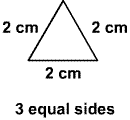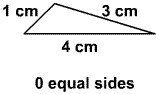Skill 23E Triangles: Isosceles, Scalene, and Equilateral Look at each triangle. Choose the name of the triangle. 1. 2. 3.isosceles trianglescalene triangle equilateral triangle isosceles trianglescalene triangle equilateral triangle isosceles trianglescalene triangle equilateral triangle